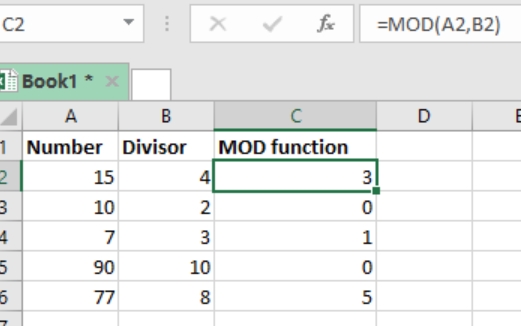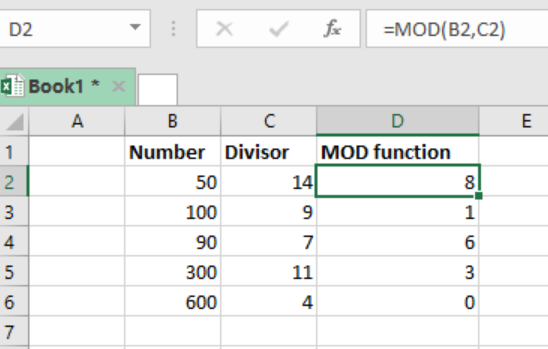Get instant live expert help with Excel or Google Sheets“My Excelchat expert helped me in less than 20 minutes, saving me what would have been 5 hours of work!”

#### Post your problem and you'll get expert help in seconds

Your message must be at least 40 characters
Our professional experts are available now. Your privacy is guaranteed.

# Excel MOD Function

We can use the Excel MOD function if we want to get the remainder of two numbers after division. While this might seem challenging, this step-by-step guide will enable you use the MOD function to get the remainder of any two numbers after division.Figure 1: Using the MOD function to get remainder after division

## Generic syntax of the formula

`=MOD (number, divisor)`

Where;

• Number – is the number to be divided
• Divisor – number to divide with

## Understanding the MOD function

This function is fundamental when it comes to getting a remainder after division.

This function is known to return a result in the same sign as the divisor.

In the event that the divisor is zero, then the MOD function will return a #DIV/0! Error.

## ExampleFigure 2: MOD function

Step 1: Enter the data in the excel sheet as shown above. Clearly indicate the number and the divisor.

Step 2: In cell D2, put the MOD function, i.e. `=MOD(B2,C2)`

Step 3: Press Enter key

Step 4: Copy the formula across the other cells.

## Other uses of Excel MOD Function

Can be used to extract time from date

Often used in formulas that deal with “every nth” value

## Instant Connection to an Expert through our Excelchat Service

Most of the time, the problem you will need to solve will be more complex than a simple application of a formula or function. If you want to save hours of research and frustration, try our live Excelchat service! Our Excel Experts are available 24/7 to answer any Excel question you may have. We guarantee a connection within 30 seconds and a customized solution within 20 minutes.

### Did this post not answer your question? Get a solution from connecting with the expert.Another blog reader asked this question today on Excelchat:## Subscribe to Excelchat.coAnother blog reader asked this question today on Excelchat: